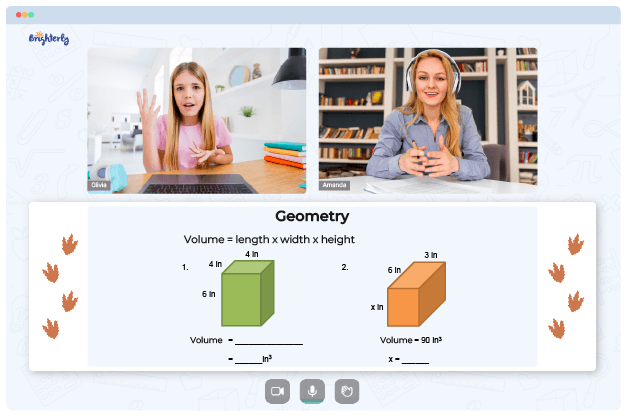# Line Plot Worksheets

Line plots are an effective method for displaying data and recognizing patterns. Line plot concepts can teach fractions, decimals, and measurement, among other math concepts. Students can practice and comprehend data analysis and representation using line plot worksheets.

Line plot worksheets grade 5 are helpful for students to comprehend data and make sense of information. Sit back as this article will give you an in-depth look at what line plot worksheets are.

## Line Plot Worksheet

Line plots show information by plotting individual interest data on a number line. Several lines and data points make up line plot worksheets. Students are instructed to position the data points on the number line correctly.

Math for Kids

Is Your Child Struggling With Math?
1:1 Online Math TutoringThey can visualize the data and connect the data points as well as recognize data trends and patterns. Students can practice solving a variety of line plot-related problems with the help of line plot worksheets. Several of the questions on these worksheets are based on interpreting word problems, making statistical inferences, and plotting data points on a line graph.

## Benefits of Line Plot Worksheets 5th Grade PDF

Line plots worksheets are used to draw several conclusions from data analysis in real-world problems. A student’s understanding of statistics will benefit more if they are well-versed in working with this subject. Line plots worksheets PDF provide students with various issues to understand the material.

Line plots 5th grade worksheets present data clearly and concisely. Students can see the data points plotted on a graph rather than trying to interpret a large table of numbers, which makes it simple for them to recognize trends and patterns. They can connect various sets of information and gain a deeper comprehension of the data.

Students are challenged to think critically and come to informed conclusions by analyzing the data and connecting various sets of information. They can utilize line plot worksheets to enhance their critical thinking abilities and develop the skills to succeed in their academic and professional endeavors.

Students can also learn about statistics and data analysis using worksheets on line plots. Mean, median, and mode are examples of the concepts these worksheets teach. They make simple data analysis fun while preparing youngsters for a career in data science, accounting, and finance.### Line Plot Worksheets PDF

Line Plot Worksheet### Line Plot Worksheets PDF

Line Plot Worksheets 5Th Grade Pdf### Line Plot Worksheets PDF### Line Plot Worksheets PDF

Line Plots Worksheets

Problems with Geometry?• Is your child having trouble grasping the concept of geometry?
• An online tutor could provide the necessary help.

Does your child struggle to master of geometry? Try learning with an online tutor.